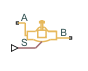# Gate Valve (TL)

Gate valve in a thermal liquid system

•Libraries:
Simscape / Fluids / Thermal Liquid / Valves & Orifices / Flow Control Valves

## Description

The Gate Valve (TL) block represents a gate valve in a thermal liquid network. The valve comprises a round, sharp-edged orifice and a round gate with the same diameter. The gate opens or closes according to the displacement signal at port S. A positive signal lifts the gate to open the valve. The diagram shows the relationship between the opening area and the net displacement of the gate.A smoothing function allows the valve opening area to change smoothly between the fully closed and fully open positions. The smoothing function reduces the abrupt opening area changes at the zero and maximum gate positions.

### Mass Balance

The mass conservation equation in the valve is

`${\stackrel{˙}{m}}_{A}+{\stackrel{˙}{m}}_{B}=0,$`

where:

• ${\stackrel{˙}{m}}_{A}$ is the mass flow rate into the valve through port A.

• ${\stackrel{˙}{m}}_{B}$ is the mass flow rate into the valve through port B.

### Momentum Balance

The momentum conservation equation in the valve is

`${p}_{A}-{p}_{B}=\frac{\stackrel{˙}{m}\sqrt{{\stackrel{˙}{m}}^{2}+{\stackrel{˙}{m}}_{cr}^{2}}}{2{\rho }_{Avg}{C}_{d}^{2}{S}^{2}}\left[1-{\left(\frac{{S}_{R}}{S}\right)}^{2}\right]P{R}_{Loss},$`

where:

• pA and pB are the pressures at port A and port B.

• $\stackrel{˙}{m}$ is the mass flow rate.

• ${\stackrel{˙}{m}}_{cr}$ is the critical mass flow rate:

`${\stackrel{˙}{m}}_{cr}={\mathrm{Re}}_{cr}{\mu }_{Avg}\sqrt{\frac{\pi }{4}{S}_{R}}.$`

• ρAvg is the average liquid density.

• Cd is the discharge coefficient.

• S is the valve inlet area.

• PRLoss is the pressure ratio:

`$P{R}_{Loss}=\frac{\sqrt{1-{\left({S}_{R}/S\right)}^{2}\left(1-{C}_{d}^{2}\right)}-{C}_{d}\left({S}_{R}/S\right)}{\sqrt{1-{\left({S}_{R}/S\right)}^{2}\left(1-{C}_{d}^{2}\right)}+{C}_{d}\left({S}_{R}/S\right)}.$`

### Energy Balance

The energy conservation equation in the valve is

`${\varphi }_{A}+{\varphi }_{B}=0,$`

where:

• ϕA is the energy flow rate into the valve through port A.

• ϕB is the energy flow rate into the valve through port B.

### Valve Opening Area

The block computes the valve opening area by using the expression

`$A=\frac{\pi {d}_{0}^{2}}{4}-{A}_{Covered}+{A}_{leak},$`

where:

• A is the valve opening area.

• Aleak is the value of the parameter.

• d0 is the valve orifice diameter.

• ACovered is the portion of the valve orifice area covered by the gate:

`${A}_{Covered}=\frac{{d}_{0}^{2}}{2}\text{acos}\left(\frac{\Delta l}{{d}_{0}}\right)-\frac{\Delta l}{2}\sqrt{{d}_{0}^{2}-{\left(\Delta l\right)}^{2}}.$`

• Δl is the net displacement of the gate center relative to the orifice center.

`$\Delta l=\left\{\begin{array}{ll}0,\hfill & \left({S}_{d}-{S}_{\mathrm{min}}\right)\le 0\hfill \\ {d}_{0},\hfill & \left({S}_{d}-{S}_{\mathrm{min}}\right)\ge {d}_{0}\hfill \\ \left({S}_{d}-{S}_{\mathrm{min}}\right),\hfill & \text{Else}\hfill \end{array}$`

• Smin is value of the Gate position when fully covering orifice parameter specified in the block dialog box.

• Sd is the gate displacement specified through physical signal input port S.

### Numerically Smoothed Displacement

When the valve is in a near-open or near-closed position, you can maintain numerical robustness in your simulation by adjusting the parameter. If the parameter is nonzero, the block smoothly saturates the gate displacement between `0` and the Valve orifice diameter parameter. For more information, see Numerical Smoothing.

## Ports

### Input

expand all

Physical signal port associated with the valve gate displacement, in m. A positive signal retracts the gate and opens the valve.

### Conserving

expand all

Thermal liquid conserving port associated with valve inlet A.

Thermal liquid conserving port associated with valve inlet B.

## Parameters

expand all

Valve open-area diameter.

Gate offset when the valve is closed. A positive, nonzero value indicates a partially open valve when the signal at port S is 0. A negative, nonzero value indicates an overlapped valve that remains closed for an initial positive displacement set by the physical signal at port .

Sum of all gaps when the valve is in the fully shut position. The block saturates smaller numbers to this value. This parameter contributes to numerical stability by maintaining continuity in the flow.

Continuous smoothing factor that introduces a layer of gradual change to the flow response when the valve is in near-open or near-closed positions.

Areas at the entry and exit ports A and B, which are used in the pressure-flow rate equation that determines the mass flow rate through the orifice.

Correction factor that accounts for discharge losses in theoretical flows.

Upper Reynolds number limit for laminar flow through the valve.

## Version History

Introduced in R2016a